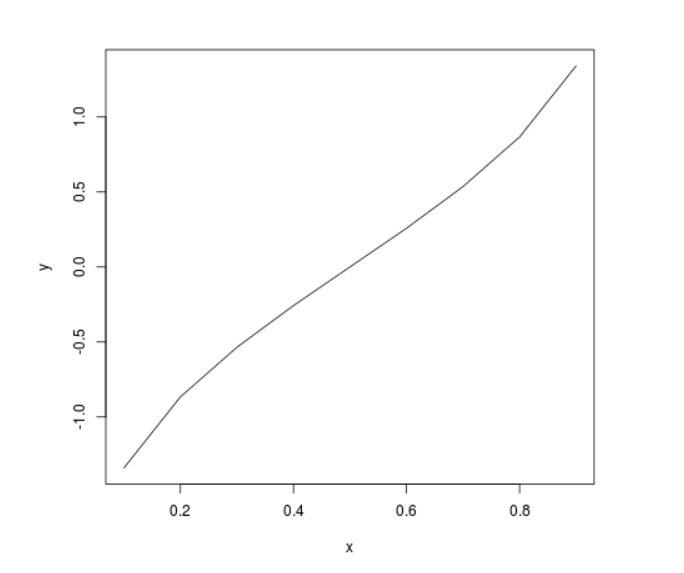GeeksforGeeks App
Open AppBrowser
Continue

# Perform the Inverse Probability Cumulative Density Analysis on t-Distribution in R Programming – qt() Function

qt() function in R Language is used to return the inverse probability cumulative density of the Student t-distribution.

Syntax: qt(x, df)
Parameters:
x: Random variable
df: Degree of Freedom

Example 1:

## Python3

 `# R Program to perform Inverse``# Cumulative Density Analysis` `# Calling qt() Function``qt(.``95``, ``10``)``qt(.``542``, ``15``)`

Output:

``` 1.812461
 0.1072654```

Example 2:

## Python3

 `# R Program to perform Inverse``# Cumulative Density Analysis` `# Creating a vector``x <``-` `seq(.``1``, .``9``, by ``=` `.``1``)` `# Calling qt() Function``y <``-` `qt(x, ``15``)` `# Plotting probability distribution graph``plot(x, y, ``type` `=``"l"``)`

Output:My Personal Notes arrow_drop_up